Test: Engineering Mathematics & General Aptitude- 1

# Test: Engineering Mathematics & General Aptitude- 1 - Civil Engineering (CE)

Test Description

## 15 Questions MCQ Test GATE Civil Engineering (CE) 2023 Mock Test Series - Test: Engineering Mathematics & General Aptitude- 1

Test: Engineering Mathematics & General Aptitude- 1 for Civil Engineering (CE) 2023 is part of GATE Civil Engineering (CE) 2023 Mock Test Series preparation. The Test: Engineering Mathematics & General Aptitude- 1 questions and answers have been prepared according to the Civil Engineering (CE) exam syllabus.The Test: Engineering Mathematics & General Aptitude- 1 MCQs are made for Civil Engineering (CE) 2023 Exam. Find important definitions, questions, notes, meanings, examples, exercises, MCQs and online tests for Test: Engineering Mathematics & General Aptitude- 1 below.
Solutions of Test: Engineering Mathematics & General Aptitude- 1 questions in English are available as part of our GATE Civil Engineering (CE) 2023 Mock Test Series for Civil Engineering (CE) & Test: Engineering Mathematics & General Aptitude- 1 solutions in Hindi for GATE Civil Engineering (CE) 2023 Mock Test Series course. Download more important topics, notes, lectures and mock test series for Civil Engineering (CE) Exam by signing up for free. Attempt Test: Engineering Mathematics & General Aptitude- 1 | 15 questions in 45 minutes | Mock test for Civil Engineering (CE) preparation | Free important questions MCQ to study GATE Civil Engineering (CE) 2023 Mock Test Series for Civil Engineering (CE) Exam | Download free PDF with solutions
 1 Crore+ students have signed up on EduRev. Have you?
Test: Engineering Mathematics & General Aptitude- 1 - Question 1

### The dimension of a pressure gradient in a fluid flow are :

Detailed Solution for Test: Engineering Mathematics & General Aptitude- 1 - Question 1

Pressure gradient= Pressure per unit length
Pressure= Force/ Area= (Mass x Acceleration)/Area =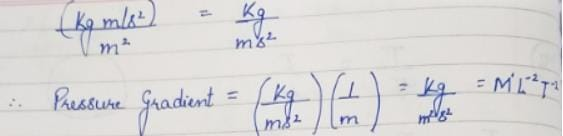Test: Engineering Mathematics & General Aptitude- 1 - Question 2

### The following Newton-Raphson iteration formula finds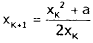Detailed Solution for Test: Engineering Mathematics & General Aptitude- 1 - Question 2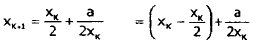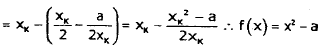Test: Engineering Mathematics & General Aptitude- 1 - Question 3

### Find the 2nd approximation to the root of the equation t4 -4t-9 = 0 between 2 and 2.5 using Bisection method.

Detailed Solution for Test: Engineering Mathematics & General Aptitude- 1 - Question 3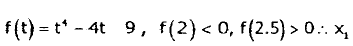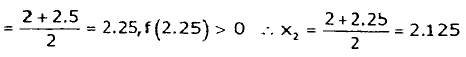Test: Engineering Mathematics & General Aptitude- 1 - Question 4

Consider two sets of vectors P and Q as

P = {(2,-1,3,2), (1,3,4,2), (3,-5,2,2)}
Q = {(.1,2,-1,0), (1,3,1,2), (4,2,1,0), (6,1,0,1)}

Which of the following is true?

Detailed Solution for Test: Engineering Mathematics & General Aptitude- 1 - Question 4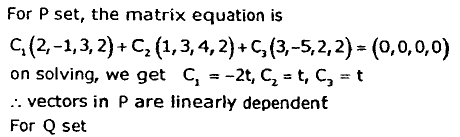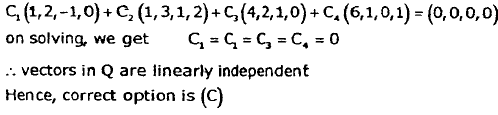Test: Engineering Mathematics & General Aptitude- 1 - Question 5

Let G1 and G2 be two edge disjoint and vertex disjoint graphs. G1 has 4 vertices and 6 edges, G2 has 6 vertices and 10 edges. Then number of edges in (G1 intersection G2) and (G1 union G2) are respectively

Detailed Solution for Test: Engineering Mathematics & General Aptitude- 1 - Question 5

Edge disjoint means no edge is common and vertex disjoint means no vertex is common.
As there is no edge is common between G1 and G2, hence number of edges in (G1 intersection G2) = 0 and number of edges in (G1 union G2) = number of edges in G1 + number of edges in G2 = 6+10 = 16

Test: Engineering Mathematics & General Aptitude- 1 - Question 6

Which of the following propositions is a tautology?

*Answer can only contain numeric values
Test: Engineering Mathematics & General Aptitude- 1 - Question 7

The minimum number of connected components of a simple graph with 20 vertices and 12 edges is

Detailed Solution for Test: Engineering Mathematics & General Aptitude- 1 - Question 7

e: number of edges = 12, n: number of vertices = 20
k: number of connected components, e > n - k , Or, 12 > 20-K ⇒ K = 8

Test: Engineering Mathematics & General Aptitude- 1 - Question 8

Which of the following graphs has a Hamiltonian circuit?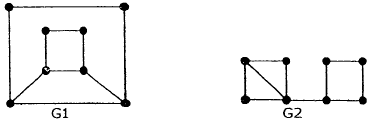Detailed Solution for Test: Engineering Mathematics & General Aptitude- 1 - Question 8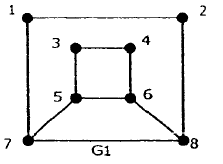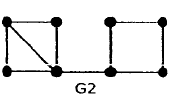G1 has a Hamiltonian circuit: 1-2-8-6-4-3-5-7-1
G2 has a bridge (an edge which, if removed, disconnects the graph), namely the middle edge of the thcee horizontal edges at the bottom of the given .drawing of G2. Whichever side of the bridge you start, you cannot, having crossed the bridge, re-cross it without visiting vertices twice. Hence you cannot complete a Hamiltonian circuit.

Test: Engineering Mathematics & General Aptitude- 1 - Question 9

The proposition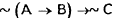logically equivalent to

Detailed Solution for Test: Engineering Mathematics & General Aptitude- 1 - Question 9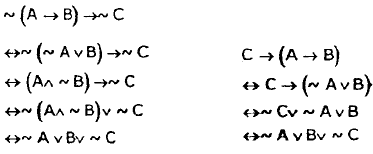Test: Engineering Mathematics & General Aptitude- 1 - Question 10

Investigate the values of λ and μ so that the equations

2x+3y+5z = 9, 7x+3y-2z = 8, 2x+3y+λ z = μ

have an infinite number of solutions.

Detailed Solution for Test: Engineering Mathematics & General Aptitude- 1 - Question 10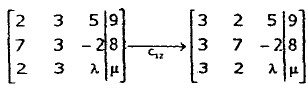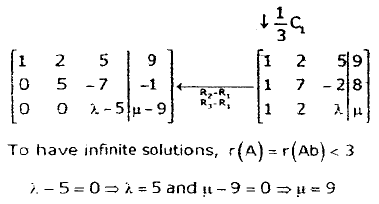Test: Engineering Mathematics & General Aptitude- 1 - Question 11

A complex (P+ iQ, P,Q : real numbers) n x n matrix A is said to be unitary if AA° = In, where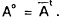If A be a unitary matrix, then which of the following is true?

Detailed Solution for Test: Engineering Mathematics & General Aptitude- 1 - Question 11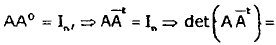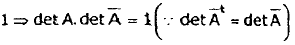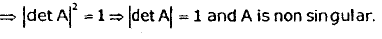*Answer can only contain numeric values
Test: Engineering Mathematics & General Aptitude- 1 - Question 12

Consider the function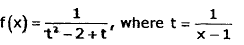At how many points, f(x) Is discontinuous?

Detailed Solution for Test: Engineering Mathematics & General Aptitude- 1 - Question 12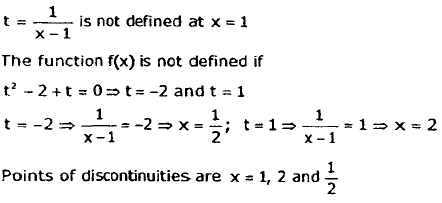Test: Engineering Mathematics & General Aptitude- 1 - Question 13

Evaluate the integral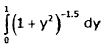Detailed Solution for Test: Engineering Mathematics & General Aptitude- 1 - Question 13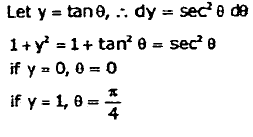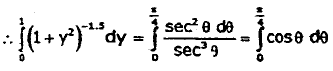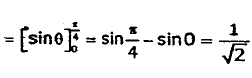Test: Engineering Mathematics & General Aptitude- 1 - Question 14

Consider the following matrix: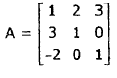Determine the eigen values of the given matrix.

Detailed Solution for Test: Engineering Mathematics & General Aptitude- 1 - Question 14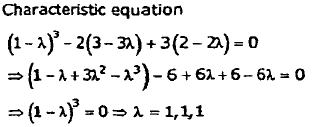Test: Engineering Mathematics & General Aptitude- 1 - Question 15

Consider the function f (x) = 2x3 - 9x2 + 12x -12

The function attains minima at x =

Detailed Solution for Test: Engineering Mathematics & General Aptitude- 1 - Question 15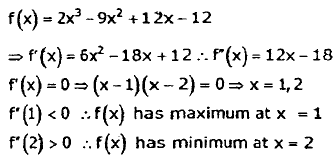## GATE Civil Engineering (CE) 2023 Mock Test Series

27 docs|296 tests
Information about Test: Engineering Mathematics & General Aptitude- 1 Page
In this test you can find the Exam questions for Test: Engineering Mathematics & General Aptitude- 1 solved & explained in the simplest way possible. Besides giving Questions and answers for Test: Engineering Mathematics & General Aptitude- 1, EduRev gives you an ample number of Online tests for practice

## GATE Civil Engineering (CE) 2023 Mock Test Series

27 docs|296 tests(Scan QR code)